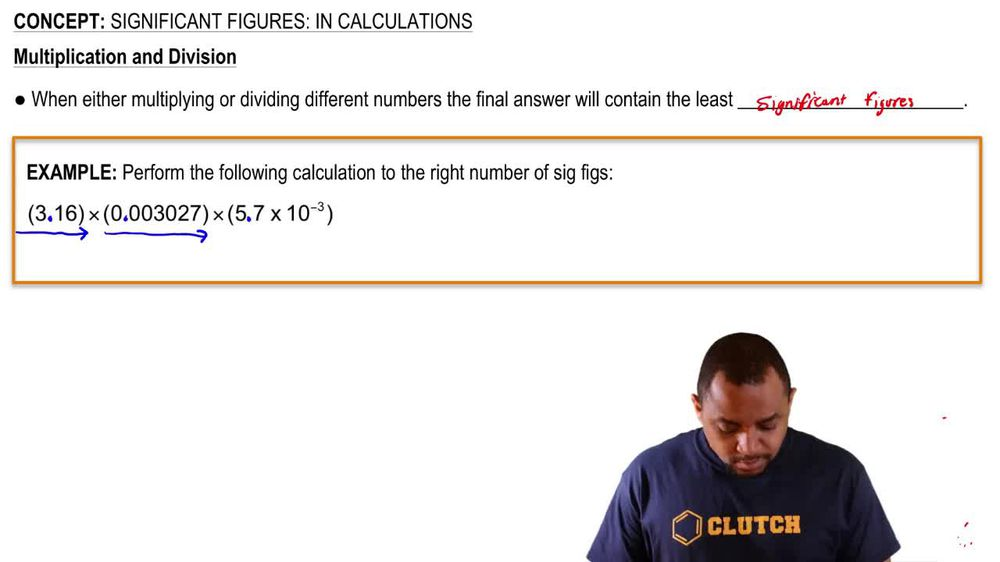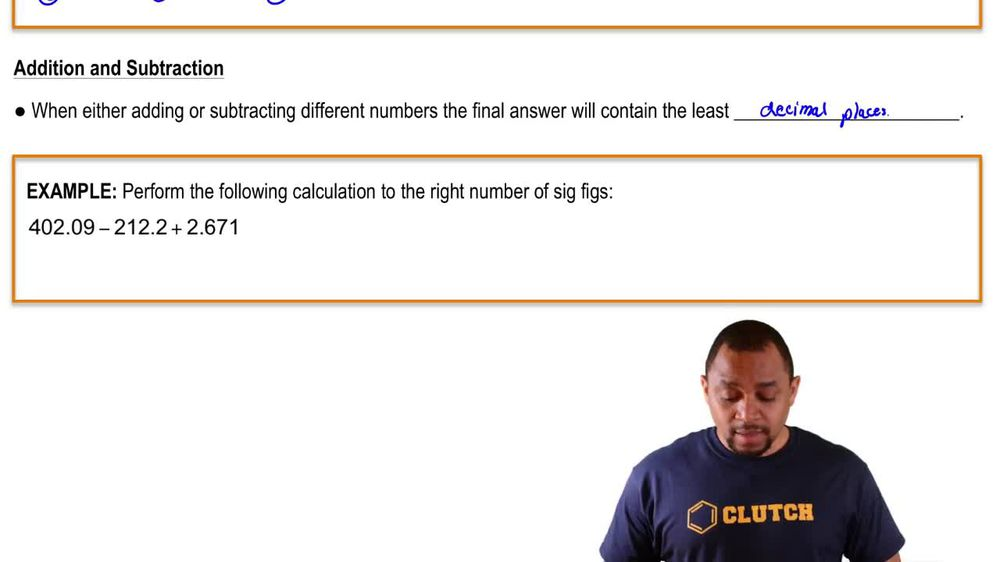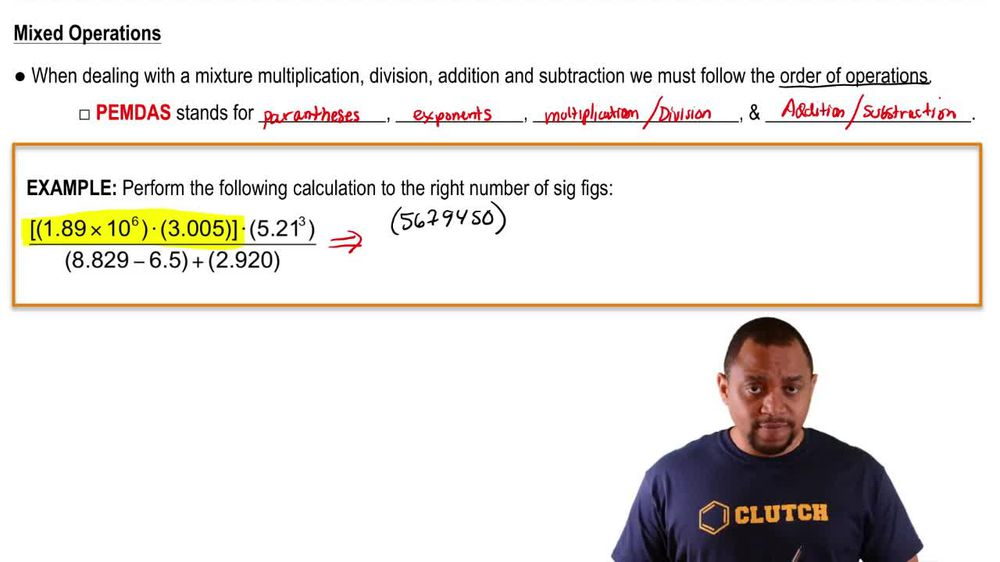Start typing, then use the up and down arrows to select an option from the list.
1. 1. Intro to General Chemistry2. Significant Figures: In Calculations
Problem

# What is the difference in mass between a nickel that weighs 4.8 g and a nickel that weighs 4.8673 g?

Relevant Solution1m
Play a video:
Hello everyone today we are being given the falling problem, calculate the mass difference between two iron substances weighing 6.39852 g and 6.39 g respectively. So an important rule to note that when we add or subtract numbers from each other we have to look for the least amount of decimal places. So we look for the lease decimal places with their answer. So when we align these two We have 6.39852 g. Subtracted by 6.39 g. Our answer is going to be 0. g. If we naturally subtracted it however, we have to look at how many significant figures there are in each Substance. So in this first substance here we have five decimal places And then the 2nd 1 We have two decimal places. Therefore our answer should only contain two decimal places. So it should only go 12 and it should round up there. Therefore this is going to round up to 0.01 g and this will be our final answer. I hope this helped until next time.